# How to denote Bra-ket notation in LaTeX?

Bra-ket or Dirac notation consists of two separate notations called bra and ket. Angle brackets and single vertical bar have been used to construct this braket notation.

## Bra and Ket notation in LaTeX

Bra notation 〈a| is bounded by a left angle bracket and vertical bar, ket notation |b〉 is bounded by a vertical bar and right angle bracket.

And multiple commands are used to represent vertical bars like vert, rvert, and mid, etc. For example

\documentclass{article}
\begin{document}
$\langle a_j | \;,\; | a_k \rangle$
$\langle m \vert \;,\; \vert n \rangle$
$\left\langle \frac{m_i}{k} \right\vert \;,\; \left\vert \frac{n_j}{k} \right\rangle$
$\left\langle \frac{p_i}{n_j} \right\vert \;,\; \left\vert \frac{q_k}{n_j} \right\rangle$
\end{document}

Output :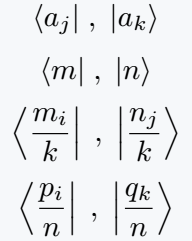## Use default command in LaTeX

There is no individual default command for bra-ket notation. So, for both angle brackets and a single bar, you have to write separate commands.

\documentclass{article}
\begin{document}
$\langle a | b \rangle$
$\langle p \vert q \rangle$
$\langle a \mid b \rangle$
$\langle \frac{m}{2} \vert \frac{n}{2} \rangle$
\end{document}

Output :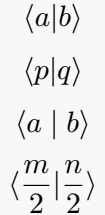When you use fraction as a variable or argument in bra- ket notation, it is very important to match the size of the variable with the size of the bra-ket notation.

\documentclass{article}
\newcommand{\braket}{\left \langle #1 \middle| #2 \right \rangle}
\newcommand{\braketmatrix}{\left \langle #1 \middle| #2 \middle| #3 \right \rangle}
\begin{document}
$\braket{e_k}{e_i}$
$\braketmatrix{\phi}{A}{\psi}$
$\braketmatrix{x}{\hat{p}}{\psi}$
$\braketmatrix{u}{\Omega^\dagger}{v}$
$\braket{\frac{m}{k}}{\frac{n}{k}}$
\end{document}

Output :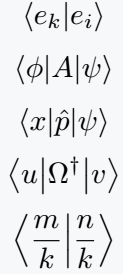You can define the same macro through the mathtools package. And the main advantage of using this package is that you do not need to use \left and right commands before the angle bracket.

The size of the symbol will be automatically adjustable.

\documentclass{article}
\usepackage{mathtools}
\DeclarePairedDelimiterX\braket{\langle}{\rangle}{#1 \delimsize\vert #2}
\begin{document}
$\braket{\phi_k}{\psi_i}$
$\braket{x}{O}$
$\braket*{\hat{X}}{\hat{Y}}$
$\braket*{\frac{e_i}{n}}{\frac{e_k}{n}}$
\end{document}

Output :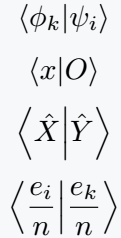## Braket package for the bra-ket notation in LaTeX

Latex has braket packages that contain two separate commands, \braket, and \Braket to represent this symbol.

Although the two commands are the same in terms of spelling, they are completely different in terms of functional work because LaTeX is case-sensitive.

In this command, the arguments are separated by a single bar and passed. For example

\documentclass{article}
\usepackage{braket}
\begin{document}
$\braket{\phi | \psi}$
$\braket{\Psi | \hat{A} | \Psi}$
$\braket{n | \frac{A}{k} | m}$
\end{document}

Output :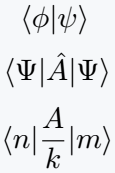If you look at the output above, you can see that the size of the symbol is fixed in the case of \braket command.

However, in the case of \Braket commands, the size of the symbol increases according to the size of the argument.

\documentclass{article}
\usepackage{braket}
\begin{document}
$\Braket{\phi | \psi}$
$\Braket{\Psi | \hat{A} | \Psi}$
$\Braket{n | \frac{A}{k} | m}$
\end{document}

Output :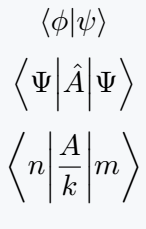## Use physics package for bra-ket notation

Different commands are defined in the physics package for each case of bra-ket notation.

And you can denote the complete notation by putting the main command bra and ket of this package side by side. For example

\documentclass{article}
\usepackage{physics}
\begin{document}
$\bra{a'}\ket{b'}$
$\bra{\alpha}\ket{\omega}$
$\bra*{\frac{\phi_i}{n}}\ket*{\frac{\phi_k}{n}}$
$\bra{\frac{\phi_i}{n}}\ket{\frac{\phi_k}{n}}$
$\bra{\phi_1+\phi_2}\ket{\psi_1+\psi_2}$
\end{document}

Output :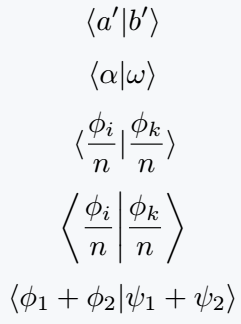Second, the best practice for this notation is to use the \braket command which is also present in the physics package.

And you can pass two arguments together for two variables.

\documentclass{article}
\usepackage{physics}
\begin{document}
% use single argument : \braket{arg}
$\braket{\phi_i}$
$\braket{k}$
% use multiple arguments : \braket{arg1}{arg2}
$\braket{x_i}{x_k}$
$\braket{\hat{A}}{O}$
% use short command like : \ip{arg1}{arg2}
$\ip{\textbf{r}}{\psi}$
$\ip{\tau}{\phi}$
\end{document}

Output :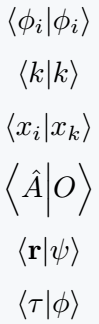If the two arguments are the same then one has to pass an argument that has been used in the above program.

And if you use the star sign with the command, the symbol size will be fixed.

\documentclass{article}
\usepackage{physics}
\begin{document}
$\braket*{m_i}{n_j}$
$\braket*{\frac{m_i}{k}}{\frac{n_j}{k}}$
\end{document}

Output :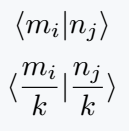Below are some more uses of the physics package for this symbol.

\documentclass{article}
\usepackage{physics}
\begin{document}
% For outerproduct : \dyad{arg1}{arg2}, \ketbra{arg1}{arg2}, and \op{arg1}{arg2}
$\dyad{a}{b}$
$\op{a}{b}$
$\ketbra{a}{b}$
% For expectation value : \expval{arg1}{arg2}, \ev{arg1}{arg2}
$\expval{A}{\Psi}$
$\ev{A}{\Psi}$
% For matrix element : \matrixel{arg1}{arg2}{arg3}, \mel{arg1}{arg2}{arg3}
$\matrixel{n}{A}{m}$
$\mel{n}{A}{m}$
\end{document}

Output :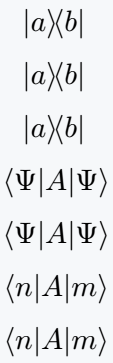#### Md Jidan Mondal

LaTeX expert with over 10 years of experience in document preparation and typesetting. Specializes in creating professional documents, reports, and presentations using LaTeX.

### 1 thought on “How to denote Bra-ket notation in LaTeX?”

1.Really helpful.. keep good helping spirits high..